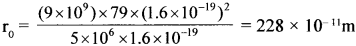# GSEB Solutions Class 12 Physics Chapter 12 Atoms

Gujarat Board GSEB Textbook Solutions Class 12 Physics Chapter 12 Atoms Textbook Questions and Answers, Additional Important Questions, Notes Pdf.

## Gujarat Board Textbook Solutions Class 12 Physics Chapter 12 Atoms

### GSEB Class 12 Physics Atoms Text Book Questions and Answers

Question 1.
Choose the correct alternative from the clues given at the end of each statement:
(a) The size of the atom in Thomson’s model …………. is the atomic size in Rutherford’s model. (much greater than/not different from/much less than)

(b) In the ground state of ………… electrons are in stable equilibrium, while in ……….. electrons always experience a net force. (Thomson’s model! Rutherford’s model.)

(c) A classical atom based on ………. is doomed to collapse. (Thomson’s model/ Rutherford’s model.)

(d) An atom has a nearly continuous mass distribution in a but has a highly non-uniform mass distribution in (Thomson’s model Rutherford’s model.)

(e) The positively charged part of the atom possesses most of the mass in ………. (Rutherford’s model/both the models.)
(a) not different from
(b) Thomson’s model; Rutherford’s model
(c) Rutherford’s model
(d) Thomson’s model; Rutherford’s model
(e) both the models

Question 2.
Suppose you are given a chance to repeat the alpha-particle scattering experiment using a thin sheet of solid hydrogen in place of the gold foil. (Hydrogen is a solid at temperatures below 14 K.) What results do you expect?
The nucleus of hydrogen contains only one proton. Its mass is 1.67 x 10-27 kg. The mass of an alpha-particle is 6.64 x 10-27 kg. When the alpha particles are incident on a thin sheet of solid hydrogen [in place of the gold foil], even in a head-on collision, the alpha-particle will not be scattered back. It is because the scattering particle is more massive than the target particle.Question 3.
What is the shortest wavelength present in the Paschen series of spectral lines?
Solution:
n = ∞, R = 1.097 x 107m-1
$$\frac {1}{λ}$$ = R $$\left[\frac{1}{3^{2}}-\frac{1}{n^{2}}\right]$$
$$\frac {1}{λ}$$ = 1.097 x 107$$\left[\frac{1}{9}\right]$$
λ = $$\frac{9}{1.097 \times 10^{7}}$$ = 8.199 x 10-7 = 8199 A

Question 4.
A difference of 2.3 eV separates two energy levels in an atom. What is the frequency of radiation emitted when the atom makes a transition from the upper level to the lower level?
Solution:
E = 2.3 eV = 2.3 x 1.6 x 10-19J
h = 6.626 x 10-34Js
E = hυ, υ = $$\frac {E}{h}$$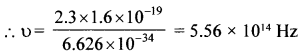Question 5.
The ground state energy of the hydrogen atom is – 13.6 eV. What are the kinetic and potential energies of the electron in this state?
KE = $$\frac{1}{8 \pi \varepsilon_{0}} \cdot \frac{\mathrm{e}^{2}}{\mathrm{r}}$$
PE = $$\frac{-1}{4 \pi \varepsilon_{0}} \frac{\mathrm{e}^{2}}{\mathrm{r}}$$
∴ KE = – $$\frac {1}{2}$$PE
Total engrty = E = KE + PE = – 13.6 eV
i.e., PE – $$\frac {1}{2}$$ PE = – 13.6 eV
PE = – 27.2 eV
∴ KE = 13.6 eV

Question 6.
A hydrogen atom initially in the ground level absorbs a photon, which excites it to the n = 4 level. Determine the wavelength and frequency of the photon.
Solution:
E = 2 eV
n = 1, E1 = – 13.6 eV
n = 4, E4 = $$\frac {-13.6}{16}$$ = -0.85 eV
∴ ∆E = E4 – E1 = – 0.85 – (- 13.6) = 12.75 eV
The hydrogen atom will go from n = 1 to n = 4 if the photon energy = 12.75 x 1.6 x 10-19J
= 20.4 x 10-19J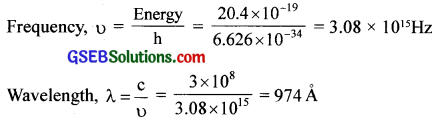Question 7.
(a) Using the Bohr’s model calculate the speed of the electron in a hydrogen atom in the n = 1, 2, and 3 levels.
(b) Calculate the orbital period in each of these levels.
Solution: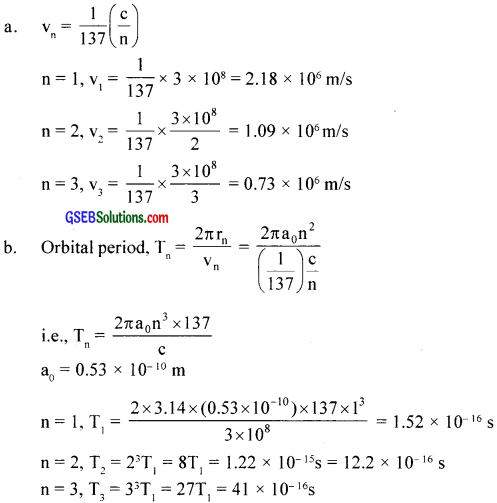Question 8.
The radius of the innermost electron orbit of a hydrogen atom is 5.3 x 10-11. What are the radii of the n = 2 and n = 3 orbits?
Solution:
rn Kn2, K =$$\frac{4 \pi \varepsilon_{0} h^{2}}{4 \pi^{2} m e^{2}}$$ = 0.53 x 10-10 = 53 x 10-11 m
n = 1, r1 K(1)2, r1 = K = 5.3 x 10-11m
n = 2, r2 = 4K = 21.2 x 10-11 m
n = 3.,r2 = 9K = 47.7 x 10-11 mQuestion 9.
A 12.5 eV electron beam is used to bombard gaseous hydrogen at room temperature. What series of wavelengths will be emitted?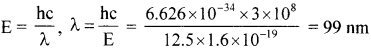On de-excitation, they may produce Balmer series, also i.e., Lyman series.

Question 10.
In accordance with Bohr’s model, find the quantum number that characteristics the earth’s revolution around the sun in an orbit of radius 1.5 x 1011 m with orbital speed 3 x 104 m/s. (Mass of earth = 6.0 x 1024 kg.)
Solution:
mvr = $$\frac {nh}{2π}$$
n = $$\frac {2πmvr}{h}$$
n = 2.56 x 1074

Question 11.
Answer the following questions, which help you to understand the difference between Thomson’s model and Rutherford’s model better.
(a) Is the average angle of deflection of a -particles by a thin gold foil predicted by Thomson’s model much less, about the same, or much greater than that predicted by Rutherford’s model?
(b) Is the probability of backward scattering (i.e., scattering of u -particles at a glass greater than 90°) predicted by Thomson’s model much less, about the same, or much greater than that predicted by Rutherford’s model?
(c) Keeping other factors fixed, it is found experimentally that for small thickness, the number of a -particles scattered at moderate angles is proportional to What clue does this linear dependence on t provide?
(d) In which model is it completely wrong to ignore multiple scattering for the calculation of the average angle of scattering of α-particles by a thin foil?
(a) Thomson’s atom model cannot explain the scattering of α -particles through large angles and particularly through 180°. It is because the light particles like electrons cannot deflect the massive a -particles through 180°.

(b) Thomson’s model predicts the backward scattering of a -particles much less than that predicted by Rutherford’s model. The reason is that in Thomson’s model, a positive charge is uniformly distributed over the whole of the nucleus instead of being concentrated in a small part.

(c) The chance of a single collision increases linearly with the number of targeted atoms and hence linearly with thickness. That the number of α -particles scattered at moderate angles is proportional to t, suggests that the scattering is predominantly due to a single collision.

(d) On the basis of Thomson’s atom model, the scattering angle of a -particles due to a single collision will be very small. It is because the mass of an atom is distributed all over it. The observed average scattering angle can be explained only by considering multiple scattering. Therefore, it is wrong to ignore multiple scattering in Thomson’s atom model.

Since the mass of the atom is concentrated in the form of the nucleus in Ruther ford’s atom model, most of the scattering comes through a single collision and multiple scattering can be ignored.Question 12.
The gravitational attraction between electron and proton in a hydrogen atom is weaker than the Coulomb attraction by a factor of about 100. An alternative way of looking at this fact is to estimate the radius of the first Bohr orbit of a hydrogen atom if the electron and proton were bound by gravitational attraction. You will find the answer interesting.
By the law of gravitation, img
i.e., me v2r = Gme mp ………..(1)
By Bohr’s condition, me vr = $$\frac {nh}{2π}$$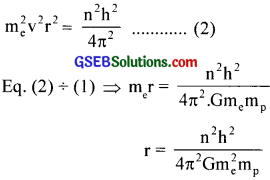For 1st orbit n = 1. h = 6.626 x 10-34 Js
G = 6.67 x 10 ‚ Nm2/kg2
me = 9 x 1011 kg, mp = 1.67 x 10-27 kg
Substituting these values. we get r = 1.21 x 10-29 m
This value of ‘r’ is much greater than the size of the universe.

Question 13.
Obtain an expression for the frequency of radiation emitted when a hydrogen atom de-excites from level n to level (n – 1). For large n, show that this frequency equals the classical frequency of revolution of the electron in the orbit.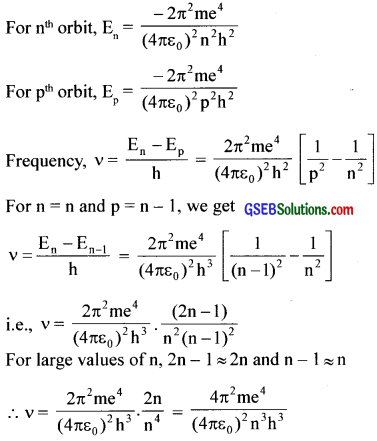Classical frequency, vc = $$\frac {v}{2πr}$$
We have mvr = $$\frac {nh}{2π}$$ ∴ v = $$\frac {nh}{2πmr}$$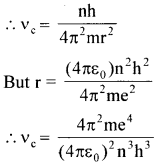Then fbr large values of n, υ = υc Hence the proof.
This is called Bohr’s correspondence principle.

Question 14.
Classically, an electron can be in any orbit around the nucleus of an atom. Then what determines the typical atomic size? Why is an atom not, say, a thousand times bigger than its typical size? The question had greatly puzzled Bohr before he arrived at his famous model of the atom that you have learned in the text. To simulate what he might well have done before his discovery, let us play as follows with the basic constants of nature and see if we can get a quantity with the dimensions of length that are roughly equal to the known size of an atom (~10-10m).

(a) Construct a quantity with the dimensions of length from the fundamental constants ‘e’, ‘m’, and ‘c’. Determine its numerical value.
(b) You will find that the length obtained in (a) is many orders of magnitude smaller than the atomic dimensions. Further, it involves ‘c’. But energies of atoms are mostly in a non-relativistic domain where ‘c’ is not expected to play any role. This is what may have suggested Bohr discard ‘c’ and look for ‘something else’ to get the right atomic size. Now, the Planck’s constant ‘h’ had already made its appearance elsewhere. Bohr’s great insight lay in recognizing that ‘h’, ‘me’, and ‘e’ will yield the right atomic size. Construct a quantity with the dimension of length from ‘h’, ‘me’, and ‘e’ and confirm that its numerical value has indeed the correct order of magnitude.
Solution:
(a) Dimensions of
(i) charge, [e] = [AT]
(ii) mass, [me] = [m1]and
(iii) [c] = [L-1T-1]
Also, dimensions of $$\left(\frac{1}{4 \pi \varepsilon_{0}}\right)$$ = $$\left[\mathrm{M}^{1} \mathrm{~L}^{3} \mathrm{~T}^{-4} \mathrm{~A}^{-2}\right]$$
Dimensions of =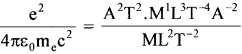= L; i.e., the dimension of length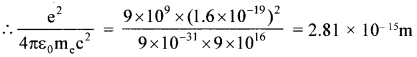This is very small as compared to the size of the atom. order of nucleus

(b) Dimensions of h – ML-2T-1; $$\left[h=\frac{\mathrm{h}}{2 \pi}\right]$$
Dimensions of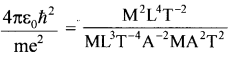= L, the dimension of length.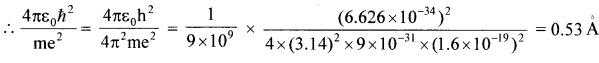This is nearly equal to the size of the atom.Question 15.
The total energy of an electron in the first excited state of the hydrogen atom is about -3.4 eV.
(a) What is the kinetic energy of the electron in this state?
(b) What is the potential energy of the electron in this state?
(c) Which of the answers above would change if the choice of the zero of potential energy is changed?
Solution:
In Bohr s model, mvr = nh and = $$\frac{\mathrm{mv}^{2}}{\mathrm{r}}$$ = $$\frac{\mathrm{Ze}^{2}}{4 \pi \varepsilon_{0} \mathrm{r}^{2}}$$
which give T = – $$\frac {1}{2}$$ mv2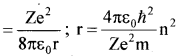These relations have nothing to do with the choice of the zero of potential energy. Now, choosing the zero of potential energy at infinity, we have V = –$$\left(\frac{\mathrm{Ze}^{2}}{4 \pi \varepsilon_{0} \mathrm{r}}\right)$$ which gives V = -2T
and E = T + V = -T
(a) The quoted value of E = -3.4 eV is based on the customary choice of zero potential energy at infinity. Using E = – T, the kinetic energy of the electron in this state is + 3.4 eV.
(b) Using V = – 2T. potential energy of the electron is = – 6.8 eV
(c) If the zero potential energy is chosen differently kinetic energy does not change. Its value is + 3.4 eV independent of the choice of the zero potential energy. The potential energy. and the total energy of the state, however, would alter if a different zero of the potential energy is chosen.

Question 16.
If Bohr’s quantisation postulate (angular momentum =$$\frac {nh}{2π}$$) is a basic law of nature, it should be equally valid for the case of planetary motion also. Why then do we never speak of quantisation of orbits of planets around the sun?
Solution:
Angular momenta associated with planetary motion are incomparably large relative to h. For example. angular momentum of the earth in its orbital motion is of the order of 1070h. In terms of Bohr’s quantisation postulate, this corresponds to a very large value of n (of the order of 1070). For such large values of n, the differences in the successive energies and angular momenta of the quantized levels of the Bohr model are so small compared to the energies and angular momenta respectively for the levels that one can, for all practical purposes, consider the levels continuous,

Question 17.
Obtain the first Bohr’s radius and the ground state energy of a moronic hydrogen atom [i.e., an atom in which a negatively charged  (µ) of mass about 207 m orbits around a proton]
Solution:
m = 207 m = 207 x 9 x 1031 kg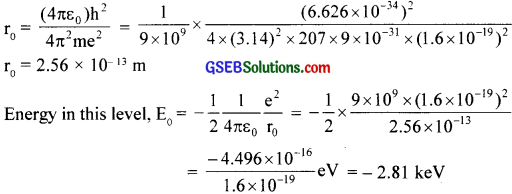Question 1.
Pick the odd one out from the following.
(a) Lyman series
(b) Paschen series
(c) Brackett series
(d) Pfund series
(e) Humphrey series
Lyman series (It is in the UV region, all the others are in the infrared region)

Question 2.
Fill in the blanks.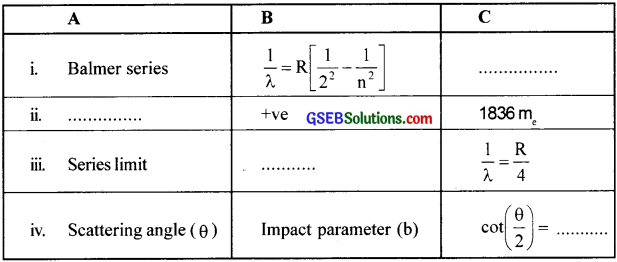i. Visible region
ii. Proton
iii. n = ∞
iv. $$\left(\frac{4 \pi \varepsilon_{0} \mathrm{~T}}{\mathrm{Ze}^{2}}\right)$$ bQuestion 3.
(a) What is impact parameter?
(b) How is it related to scattering angle?
(a) Impact parameter
(b) of an a -particle is the perpendicular distance of the velocity vector of the α-particle from the centre of the nucleus when it is away from the nucleus.
(b) Scattering angle = θ, impact parameter = b
cot $$\frac {θ}{2}$$ = $$\left(\frac{4 \pi \varepsilon_{0} \mathrm{~T}}{\mathrm{Ze}^{2}}\right)$$ b

Question 4.
What is the Bohr quantization condition for the angular momentum of an electron in the second orbit?
mvr = $$\frac {nh}{2π}$$ = $$\frac {2h}{2π}$$ = $$\frac {h}{π}$$

Question 5.
(a) For the calculation of the average angle of scattering of α -particles by a thin foil, why multiple scattering cannot be ignored in the Thomson model?
(b) Name the model in which it can be ignored
(c) The majority of -particles pass through gases with no deflections. To what conclusion about the atomic structure does this observation lead?
(a) Positive charge is uniformly distributed in the whole atom of Thomson’s model. If an only a single collision is considered, it cannot cause large deflection, so multiple scattering is considered to calculate the average angle of scattering. Thus multiple scattering cannot be ignored in Thomson’s model.
(b) It can be ignored in Rutherford’s model because scattering is mainly because of a single collision in this model.
(c) This observation leads to the conclusion that most of the space in the atom is empty.

Question 6.
For scattering by an ‘inverse square field’ (such as that produced by a charged nucleus in the Rutherford c model), the relation between impact parameter h and the scattering angle θ is given by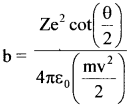(a) What Ls the scattering angle for b = 0?
(b) For a given impact parameter b, does the angle of deflection increase or decrease with increasing energy?
(c) What is the impact parameter at which the scattering angle is 90° for Z = 79 and initial energy of 10 MeV?
(d) Why is ft that the muss of the nucleus does not enter the formula above hut the charge does?
(e) For the given energy of the projectile, does the scattering angle increase or decrease with a decrease in impact parameter?
(a) For b = 0, we get cot $$\frac {θ}{2}$$ = 0. It means, $$\frac {θ}{2}$$ = 90° or θ = 180°
(b) Increase in b means increase in cot $$\frac {θ}{2}$$ i.e., decrease in θ
(c) U sing b = $$\frac{\mathrm{Ze}^{2} \cot \frac{\theta}{2}}{4 \pi \varepsilon_{0} \mathrm{E}}$$ we get b = 1.1 x 10-14m
(e) Decrease in h means decrease in cot $$\frac {θ}{2}$$ increase in scattering angle θU sing r0 = $$\frac{1}{4 \pi \varepsilon_{0}} \frac{Z \mathrm{e}^{2}}{\mathrm{E}}$$ we get